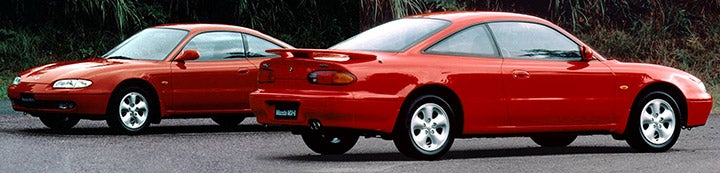1 - 1 of 1 Posts

#### 91gd626turbo

·
##### Registered
Joined
·
1,334 Posts
Discussion Starter · ·
Two solar panels as seen in the picture, one big and one small.

Large one is used but likenew in box, small one is brand new in box.

Here are the specs:

LARGE PANEL:

Dimensions = 25cm x 40cm
Brand = Solarex (Company Now Called: BP Solar)
Model = MSX10
Frame Colur = Black

STC @ 1000 W.m-2 1.5 CELL T (25 Degrees)
Peak Power (Pmax) = 10.2 W
Open Circuit Voltage (Voc) = 21.3 V
Short Circuit Current (Isc) = 0.64 A
Vpmax = 16.7 V
Ipmax = 0.61 A

STC @ 800 W.m-2 1.5 CELL T (49 Degrees)
Peak Power (Pmax) = 7.4 W
Ipmax = 0.5 A

Maximum Series Fuse = 1.5 A
Maximum System Operating Voltage = 30 V

SMALL PANEL:

Dimensions = 27cm x 24.5cm
Brand = BP Solar
Model = SX305M
Frame Colur = Silver

Peak Power (Pmax) = 4.5 W
Warranted Minimum Pmax = 4 W
Voltage (Vmp) = 16.5 V
Current (Imp) = 0.27 A
Open Circuit Voltage (Voc) = 20.5 V
Short Circuit Current (Isc) = 0.3 A
Maximum Series Fuse = 1 A

Price:

\$80 FREE SHIPPING

1 - 1 of 1 Posts